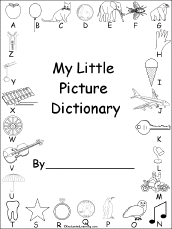Printable Picture DictionariesShort, printable picture dictionaries - each with 26 pages in PDF format. One has a word for each picture, one has room for the student to write each word, and one has a missing letter in each word for the student to fill in.K-3 Theme Pages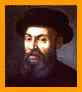Famous ExplorersFrom Armstrong to Vespucci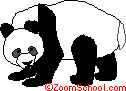Animal PrintoutsHundreds of animal coloring pages with labels and information.

• To browse through the dictionary, click on a letter in the alphabet at the top of the window and you'll see a page of words that start with that letter.
• illustrated dictionary entries! Each word is used in a meaningful example sentence. Most entries have links to a related web site. Just click on an underlined word (or its accompanying picture), and you'll link to a related page.
• The LITTLE EXPLORERSTM picture dictionary makes surfing the Internet very easy. A picture-dictionary format is used to link to hundreds of carefully-chosen child-friendly sites around the world. Since the pictures are links, even pre-readers can surf with a minimum of help and guidance from their favorite adult. Older kids can use Little Explorers as a school reference.
• This is the English version; just click to use the different versions:

• Have fun exploring and learning!
• We were a semi-finalist in the 1998 GII awards (Global Information Infrastructure awards) in the children's category.
• Listed on the BBC education web index
• By Jeananda ColDictionary Classroom Activities:Scavenger HuntsSearch for words in the dictionary to answer questions - dictionary scavenger hunts!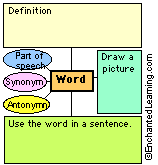Vocabulary Map PrintoutsVocabulary maps are graphic organizers that can be useful in helping a student learn new vocabulary words. Labeled cells in the printouts prompt the student to write the word, its definition, its part of speech, a synonym, an antonym, do a drawing that illustrates the word's meaning, and write a meaningful sentence containing the word.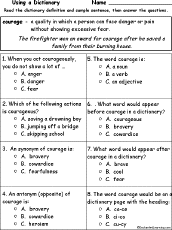Dictionary Definition WorksheetsRead the definition of a word, then answer multiple-choice questions about the word.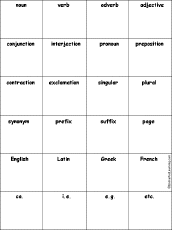Dictionary Term AbbreviationsWrite the abbreviations for common dictionary terms, including noun, verb, adjective, Greek, etc. Or go to the answers.

Countries with the most English-language speakers:
Country Number of English Speakers (approximate; X=1,000,000 people)
USA 237.7 million X X X X X X X X X X X X X X X X X X X X X X X X X X X X X X X X X X X X X X X X X X X X X X X X X X X X X X X X X X X X X X X X X X X X X X X X X X X X X X X X X X X X X X X X X X X X X X X X X X X X X X X X X X X X X X X X X X X X X X X X X X X X X X X X X X X X X X X X X X X X X X X X X X X X X X X X X X X X X X X X X X X X X X X X X X X X X X X X X X X X X X X X X X X X X X X X X X X X X X X X X X X X X X X X X X X X X X X X X X X X X X X X X X X X X X X X X X X X X X X X X X X
UK 58 million X X X X X X X X X X X X X X X X X X X X X X X X X X X X X X X X X X X X X X X X X X X X X X X X X X X X X X X X X X
Canada 18 million X X X X X X X X X X X X X X X X X X
Australia 15.5 million X X X X X X X X X X X X X X X
Ireland 3.72 million X X X X
South Africa 3.7 million X X X
New Zealand 3.3 million X X
Jamaica 2.5 million X X
Trinidad and Tobago 1.2 million X
Guyana 0.8 million X